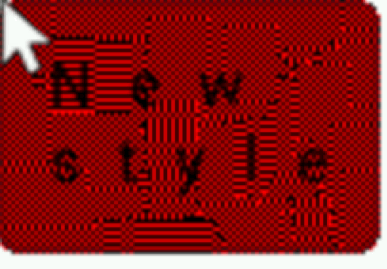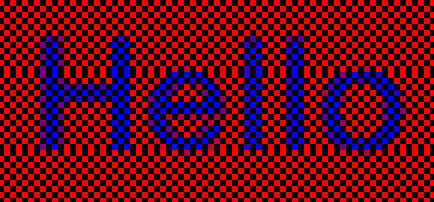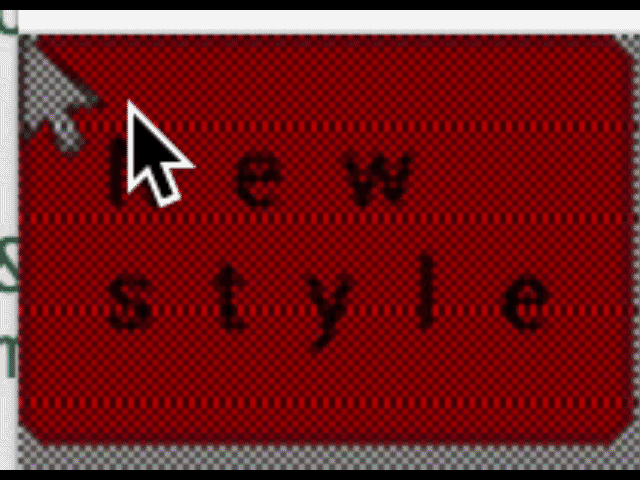# Get absolute pixel address in set_px_cb? (halftone fill)

## Description

I am implementing a halftone fill capability (for monochrome displays).
In lvgl 5.3 I did this by modifying the sw_color_fill function. halftone issue

In 5.3 the absolute address was passed in via mem_area
In 6.0 this function has changed parameters, how do I retrieve the absolute address of each pixel?

IMXRT1052

### What do you want to achieve?

Halftone fill function

### What have you tried so far?

Modifying sw_color_file with the following code.

## Code to reproduce

Here is part of the sw_color_file code I modified (this is using a relative address, row or col, not absolute)

The code block(s) should be formatted like:

``````static void sw_color_fill(lv_color_t * mem, lv_coord_t mem_width, const lv_area_t * fill_area, lv_color_t color, lv_opa_t opa)
{
/*Set all row in vdb to the given color*/
lv_coord_t row;
lv_coord_t col;

lv_disp_t * disp = lv_refr_get_disp_refreshing();
if(disp->driver.set_px_cb) {
for(col = fill_area->x1; col <= fill_area->x2; col++) {
for(row = fill_area->y1; row <= fill_area->y2; row++) {
disp->driver.set_px_cb(&disp->driver, (uint8_t *)mem, mem_width, col, row, color, opa);
}
}
} else {
mem += fill_area->y1 * mem_width; /*Go to the first row*/

/*Run simpler function without opacity*/
if(opa == LV_OPA_COVER) {
...
}
else if(opa == LV_OPA_HALFTONE)
{
//printf("half: %d\n", opa);
/*Fill the first two rows with halftone pattern*/
for(col = fill_area->x1; col <= fill_area->x2; col++)
{
if(col % 2 == 0)
{
mem[col] = color;
}
else
{
mem[col] = LV_COLOR_BLACK;
}
}
lv_color_t * mem_first = &mem[fill_area->x1];
mem += mem_width;

for(col = fill_area->x1; col <= fill_area->x2; col++)
{
if(col % 2 == 0)
{
mem[col] = LV_COLOR_BLACK;
}
else
{
mem[col] = color;
}
}

/*Copy the correct row to all other rows*/
lv_color_t * mem_second = &mem[fill_area->x1];
lv_coord_t copy_size   = (fill_area->x2 - fill_area->x1 + 1) * sizeof(lv_color_t);
mem += mem_width;

for(row = fill_area->y1 + 2; row <= fill_area->y2; row++) {
if(row % 2 == 0)
{
memcpy(&mem[fill_area->x1], mem_first, copy_size);
}
else
{
memcpy(&mem[fill_area->x1], mem_second, copy_size);
}
mem += mem_width;
}
}
/*Calculate with alpha too*/
else {
...
``````

## Screenshot and/or video

Here is a refined condition block for halftone fill, still not working, but closer:

``````		// calculate halftone fill
else if (opa == LV_OPA_HALFTONE)
{
lv_color_t *mem_curr = mem;
for (row = fill_area->y1; row <= fill_area->y2; row++) {
for (col = fill_area->x1; col <= fill_area->x2; col++) {
if ((int) (mem_curr) % 4 == 0) {
mem[col] = LV_COLOR_BLACK;
} else {
mem[col] = color;
}
mem_curr++;
}
mem += mem_width;
}
}
``````

The address becomes relative because of the varying mem_width, which causes a redraw to mismatch depending on the area.

Here is a screenshot to demonstrate, the mismatches are where the mouse pointer caused a redraw of the area:

Create your manual halttone function and regist to
`disp->driver.set_px_cb` instead of `modifying sw_color_fill(...)`

such as

``````void halftone_set_px_cb(struct _disp_drv_t * disp_drv,
uint8_t * buf, lv_coord_t buf_w,
lv_coord_t x, lv_coord_t y,
lv_color_t color, lv_opa_t opa)
{
// (x, y)  is current pixel
}
``````

OK, I tried that as well… it still looks like x and y are relative to the area being filled, not the entire screen.

``````void halftone_set_px_cb(struct _disp_drv_t *disp_drv, uint8_t *buf,
lv_coord_t buf_w, lv_coord_t x, lv_coord_t y, lv_color_t color,
lv_opa_t opa) {

bool scr_transp = false;
#if LV_COLOR_DEPTH == 32 && LV_COLOR_SCREEN_TRANSP
scr_transp = disp->driver.screen_transp;
#endif

lv_color_t *vdb_px_p = buf;
vdb_px_p += y * buf_w + x;

if (scr_transp == false) {
if (opa == LV_OPA_COVER) {
*vdb_px_p = color;
} else if (opa == LV_OPA_HALFTONE) {
printf("x:%d y:%d\n", x, y);
if (x % 2 == 0) {
if (y % 2 == 0) {
*vdb_px_p = LV_COLOR_BLACK;
} else {
*vdb_px_p = color;
}
} else {
if (y % 2 != 0) {
*vdb_px_p = LV_COLOR_BLACK;
} else {
*vdb_px_p = color;
}
}
} else {

*vdb_px_p = lv_color_mix(color, *vdb_px_p, opa);
}
} else {
#if LV_COLOR_DEPTH == 32 && LV_COLOR_SCREEN_TRANSP
*vdb_px_p = color_mix_2_alpha(*vdb_px_p, (*vdb_px_p).ch.alpha, color, opa);
#endif
}
}
``````Parameters that are sent to the `sw_color_fill(...)` function,
they are already relative area, not entire screen.
Then the area in your manual `halftone_set_px_cb()` is relative area too.

I’ve tried on my system color16bit by the following code.
(Not sure on the monochrome system is worked or not. )

## Code

``````void halftone_set_px_cb(struct _disp_drv_t * disp_drv, uint8_t * buf, lv_coord_t buf_w, lv_coord_t x, lv_coord_t y,
lv_color_t color, lv_opa_t opa)
{
//  uint32_t* c = (uint32_t*)&buf[(x+y*buf_w)*4]; // color32bit
uint16_t* c = (uint16_t*)&buf[(x+y*buf_w)*2];   // color16bit
//  uint8_t* c = (uint8_t*)&buf[(x+y*buf_w)];     // color8bit

if( (x % 2 == 0 && y % 2 == 1) ||(x % 2 == 1 && y % 2 == 0) )
color = LV_COLOR_BLACK;

if( opa == LV_OPA_COVER) {
*c = color.full;
}else{
lv_color_t color_buf; color_buf.full = *c;
lv_color_t color_mix = lv_color_mix( color, color_buf, opa);
*c = color_mix.full;
}
}
``````

## ResultThanks for trying it!
It looks like it still suffers from the same problem, without the absolute coords, there is no guarantee that a pixel will be the same color every time and you get mismatches, on the edges of the buffer, during redraw, etc.OK, I used this method to get back to the absolute coordinates in the callback:

``````else if (opa == LV_OPA_HALFTONE)
{
lv_disp_t *disp = lv_refr_get_disp_refreshing();
lv_disp_buf_t *vdb = lv_disp_get_buf(disp);
//printf("abs x:%d y:%d\n", vdb->area.x1 + x, vdb->area.y1 + y);
bool x_even = (vdb->area.x1 + x) % 2;
bool y_even = (vdb->area.y1 + y) % 2;

if ((x_even && y_even) || (!x_even && !y_even))
{
*vdb_px_p = LV_COLOR_BLACK;
}
else
{
*vdb_px_p = color;
}
}
``````2 Likes

@TridentTD I noticed a strange effect with the last image you posted:It looks like every few lines the halftone fill is not being alternated correctly.

You can see it clearly in this picture:Notice the two vertical pixels on the 11th and 12th rows.

That is also because of the relative coords (I assume) where the edges of the buffer are. You can see the same effect a bit in my animation before I fixed it with absolute coords.

Also, is this something that others would be interested in adding to the core library?

It is a fairly common way of adding variation to a monochrome display. I also added different halftone sizes, so instead of 1x1 squares, using larger 2x2, etc.
Ideally the OPA enumeration would be changed or another enumeration would be added for halftone, right now I “hijack” a couple of opacity values to designate halftone fill types, which requires changes to lv_color.h

@embeddedt
Yes, because of point(x,y) is relative point as @jking said.

This point(x,y) must turn to the absolute point by @jking’s code.

@jking
Because of the first parameter of callback is `disp_drv_t`.
Another technic for getting absolute point.

``````  bool x_even = ( disp_drv->buffer->area.x1 + x )%2;
bool y_even = ( disp_drv->buffer->area.y1 + y )%2;
``````